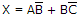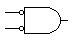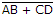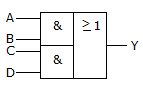# Digital Electronics - Combinational Logic Analysis

### Exercise :: Combinational Logic Analysis - Filling the Blanks

1.

Assume you have A, B, C, and D available but not their complements. The minimum number of 2-input NAND gates required to implement the equationis ________.

 A. 3 B. 4 C. 5 D. 6

Answer: Option C

Explanation:

No answer description available for this question. Let us discuss.

2.

The symbol shown represents a(n) ________.A. AND gate B. OR gate C. NAND gate D. NOR gate

Answer: Option D

Explanation:

No answer description available for this question. Let us discuss.

3.

A gate can drive a number of load gate inputs up to its specified ________.

 A. supply voltage B. noise margin C. fan-in D. fan-out

Answer: Option D

Explanation:

No answer description available for this question. Let us discuss.

4.

The expressioncan be directly implemented using only ________.

 A. an XOR gate B. an XNOR gate C. an AOI circuit D. three 2-input NAND gates

Answer: Option C

Explanation:

No answer description available for this question. Let us discuss.

5.

The symbol shown represents ________.A. AND-OR logic B. AOI logic C. XOR gate D. XNOR gate

Answer: Option A

Explanation:

No answer description available for this question. Let us discuss.

#### Current Affairs 2021

Interview Questions and Answers